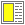# Exercise solution: Every second element of a list

```;; Return every second element of list, starting with the first element.
;; This function is useful to extract the keys or values of a property list.
(define (every-second-element lst)
(cond ((null? lst) '())
((null? (cdr lst)) (list (car lst)))
(else (cons (car lst) (every-second-element (cddr lst))))))```